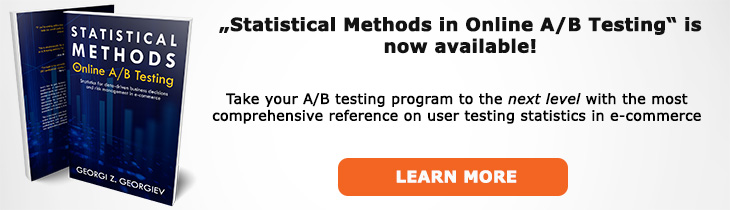# What does "Point Hypothesis" mean?

Definition of Point Hypothesis in the context of A/B testing (online controlled experiments).

## What is a Point Hypothesis?

In hypothesis testing a point hypothesis is a hypothesis that covers only a single value from the possible parameter values. For example, if the entire parameter space covers -∞ to +∞ a point hypothesis could be zero (0). It could be any other number as well, such 1, 2 or 3,1245.

In a Null Hypothesis Statistical Test only the null hypothesis can be a point hypothesis. The alternative hypothesis is always a composite hypothesis: either one-sided hypothesis if the null is composite or a two-sided one if the null is a point null.

Testing a point null is not often practical in A/B testing where we are usually interested in detecting and estimating effects in only one direction: either an increase in conversion rate or average revenue per user, or a decrease in unsubscribe events would be of interest and not its opposite. In fact, running a test so long as to detect a statistically significant negative outcome can result in significant business harm.

## Articles on Point Hypothesis

Like this glossary entry? For an in-depth and comprehensive reading on A/B testing stats, check out the book "Statistical Methods in Online A/B Testing" by the author of this glossary, Georgi Georgiev.## Glossary Index by Letter

Select a letter to see all A/B testing terms starting with that letter or visit the Glossary homepage to see all.